Courses

# Strain Analysis Mechanical Engineering Notes | EduRev

## Mechanical Engineering : Strain Analysis Mechanical Engineering Notes | EduRev

The document Strain Analysis Mechanical Engineering Notes | EduRev is a part of the Mechanical Engineering Course Machine Design.
All you need of Mechanical Engineering at this link: Mechanical Engineering

Introduction

No matter what stresses are imposed on an elastic body, provided the material does not rupture, displacement at any point can have only one value. Therefore the displacement at any point can be completely given by the three single valued components u, v and w along the three co-ordinate axes x, y and z respectively. The normal and shear strains may be derived in terms of these displacements.

Normal strains

Consider an element AB of length δx (figure-2.3.2.1). If displacement of end A is u, that of end B is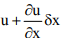. This gives an increase in length of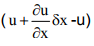and therefore the strain in x-direction is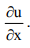Similarly, strains in y and z directions are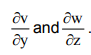.Therefore, we may write the three normal strain components as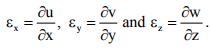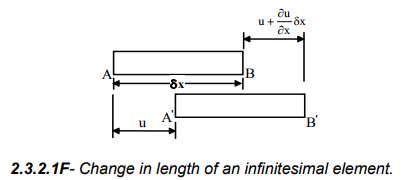Shear strain

In the same way we may define the shear strains. For this purpose consider an element ABCD in x-y plane and let the displaced position of the element be A′B′C′D′ ( Figure-2.3.3.1). This gives shear strain in xy plane as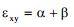where α is the angle made by the displaced line B′C′ with the vertical and β is the angle made by the displaced line A′D′ with the horizontal. This gives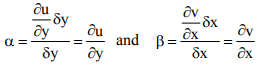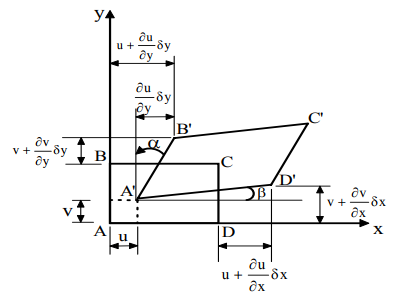2.3.3.1F- Shear strain associated with the distortion of an infinitesimal element.

We may therefore write the three shear strain components as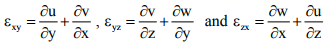Therefore, the complete strain matrix can be written as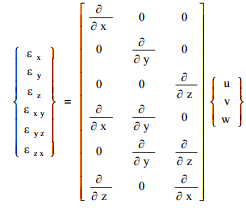Constitutive equation

The state of strain at a point can be completely described by the six strain components and the strain components in their turns can be completely defined by the displacement components u, v, and w. The constitutive equations relate stresses and strains and in linear elasticity we simply have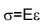where E is  modulus of elasticity. It is also known that σx produces a strain of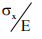in xdirection,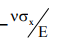in y-direction and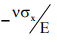in z-direction. Therefore we may write the generalized Hooke’s law as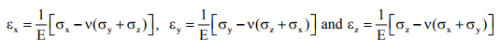It is also known that the shear stress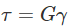where G is the shear modulus and γ is shear strain. We may thus write the three strain components as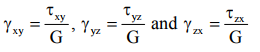In general each strain is dependent on each stress and we may write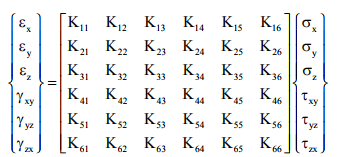For isotropic material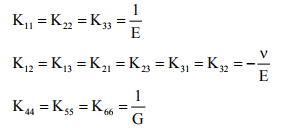Rest of the elements in K matrix are zero.

On substitution, this reduces the general constitutive equation to equations for isotropic materials as given by the generalized Hooke’s law. Since the principal stress and strains axes coincide, we may write the principal strains in terms of principal stresses as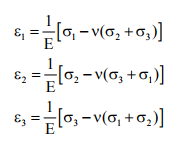From the point of view of volume change or dilatation resulting from hydrostatic pressure we also have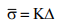where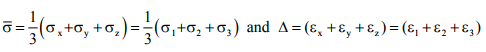These equations allow the principal strain components to be defined in terms of principal stresses. For isotropic and homogeneous materials only two constants viz. E and ν are sufficient to relate the stresses and strains. The strain transformation follows the same set of rules as those used in stress transformation except that the shear strains are halved wherever they appear.

Relations between E, G and K

The largest maximum shear strain and shear stress can be given by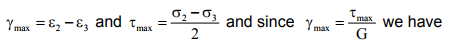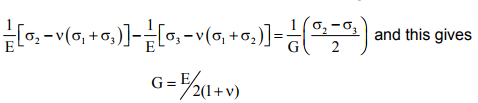Considering now the hydrostatic state of stress and strain we may write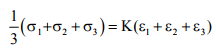Substituting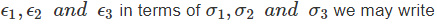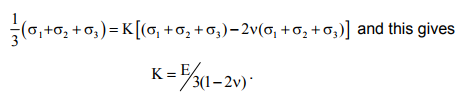Elementary thermoelasticity

So far the state of strain at a point was considered entirely due to applied forces. Changes in temperature may also cause stresses if a thermal gradient or some external constraints exist. Provided that the materials remain linearly elastic, stress pattern due to thermal effect may be superimposed upon that due to applied forces and we may write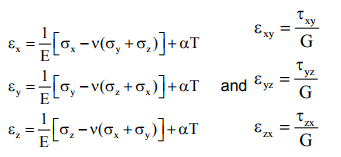It is important to note that the shear strains are not affected directly by temperature changes. It is sometimes convenient to express stresses in terms of strains. This may be done using the relation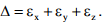Substituting the above expressions for εx , εy and εz  we have.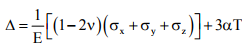and substituting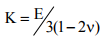we have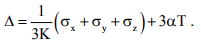Combining this with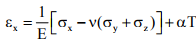we  have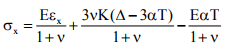Substituting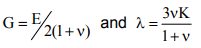we may write the normal and shear stresses as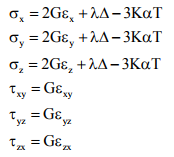These equations are considered to be suitable in thermoelastic situations.

Offer running on EduRev: Apply code STAYHOME200 to get INR 200 off on our premium plan EduRev Infinity!

## Machine Design

57 videos|71 docs|102 tests

,

,

,

,

,

,

,

,

,

,

,

,

,

,

,

,

,

,

,

,

,

;